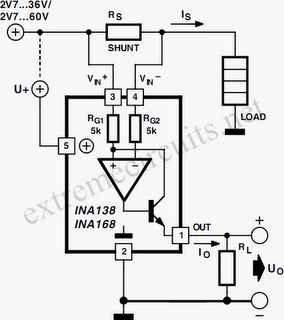# Learning Electronics

Learn to build electronic circuits

# High Side Current Measurements

It’s always a bit difficult to measure the current in the positive lead of a power supply, such as a battery charger. Fortunately, special ICs have been developed for this purpose in the last few years, such as the Burr-Brown INA138 and INA168. These ICs have special internal circuitry that allows their inputs to be connected directly to either end of a shunt resistor in the lead where the current is to be measured. The shunt is simply a low-value resistor, across which a voltage drop is measured whenever a current ﬂows. This voltage is converted into an output current Io by the IC.

This current can be used directly, or it can be converted into a voltage by means of a load resistor RL. In the latter case, the ‘ﬂoating’ measurement voltage across the shunt is converted into a voltage with respect to earth, which is easy to use. The value of RL determines the gain. A value of 5 kΩ gives 1×, 10 kΩ gives 2×, 15 kΩ gives 3× and so on. It all works as follows. Just like any opamp, this IC tries to maintain the same potential on its internal plus and minus inputs. The minus input is connected to the left-hand end of the shunt resistor via a 5-kΩ resistor.When a current flows through the shunt, this voltage is thus lower than the voltage on the plus side. However, the voltage on the plus input can be reduced by allowing a small supplementary current to flow through T1. The IC thus allows T1 to conduct just enough to achieve the necessary lower voltage on the plus input. The current that is needed for this is equal to Vshunt / 5 kΩ. This transistor current leaves the IC via the output to which RL is connected. If the value of RL is 5 kΩ, the resulting voltage is exactly the same as Vshunt. The IC is available in two versions.

The INA138 can handle voltages between 2.7 and 36 V, while the INA168 can work up to 60 V. The supply voltage on pin 5 may lie anywhere between these limits, regardless of the voltage on the inputs. This means that even with a supply voltage of only 5 V, you can make measurements with up to 60 V on the inputs! However, in most cases it is simplest to connect pin 5 directly to the voltage on pin 3. Bear in mind that the value of the supply voltage determines the maximum value of the output voltage. Also, don’t forget the internal base-emitter junction voltage of T1 (0.7 V), and the voltage drop across the shunt also has to be subtracted.